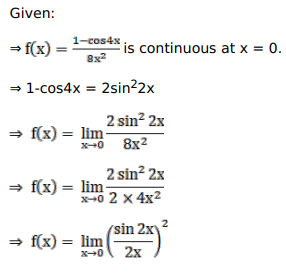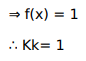# Solve this following

Question:

Mark $(\sqrt{)}$ against the correct answer in the following:

If the function $f(x)=\left\{\begin{array}{c}\frac{1-\cos 4 x}{8 x^{2}}, x \neq 0 \\ k, x=0\end{array}\right.$ is continuous at $x=0$ and then $k=?$

A. 1

B. 2

C. $\frac{1}{2}$

D. $\frac{-1}{2}$

Solution: